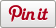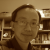# Chaos game - square fractal

•• Sharebar
•Chaos game is a mathematical way to generate fractals. It has a simple algorithm, which (1) starts from a radom point with a polygon, then (2) randomly selects a vertice, (3) plots a new point midway between the starting point and the selected vertice. The ploted mid point then becomes the step (1) and reiterates the steps as many times as you like to generate a geometric figure. The example below in this blog uses a square as the polygon to generate a chaos game square fractal.

The chaos game sqaure fractal below has two animations (i.e., spin and travel)  applied for viewing. You can also navigate or rotate in the 3D space for your preferred viewpoint.

Perhaps the most famous one is that if you start with a trianlge, you will get the Sierpiński triangle. The interesting thing is that you can start from any polygon (I have not tried concave polygons) such as regular or irregular, and you can move midway or other distance such as one third to generate different fractals. This Wikipedia page demonstrates some examples of chaos game fractals. Below is the Logo program you may want to try in VRMath2 Editor.

Further, this chaos game rule can be applied to 3D. This page shows some fractals I presented in ATCM Conference in 2017. You can try these fractals in the VRMath2 Editor by openning the logo grograms in Example --> logo --> fractal folder.